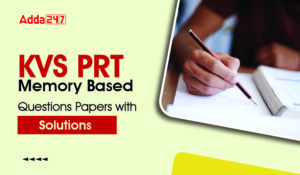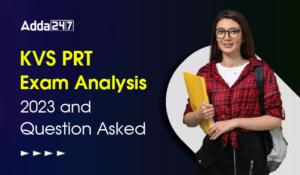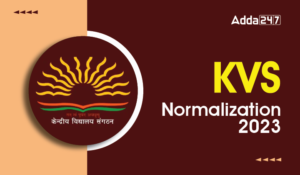Latest Teaching jobs   »   Maths Pedagogy Questions for All Teaching...

# Maths Pedagogy Questions for All Teaching Exam :8th February 2019

Dear Students!!! There is most general as well as a scoring section in all the competitive entrance examinations in the teaching field i.e “Mathematics”.Because in this section only one thing is work i.e your accuracy and that could be nourished with the daily practice. So, for this, we are providing you the daily quiz for all teaching exams i.e CTET Exam 2019, DSSSB ,KVS,STET Exam.

GET 5% DISCOUNT on CTET Prime :

Use This Code TEACH5

Q1. When a grade III teacher asked her students to describe an angle, a student described it as : ‘a place where two lines meet’ This student:
(a) Has not understood that angle is the region between the two lines
(b) Needs to learn to definition of angles by-heart
(c) Needs help in understanding geometry and geometrical concepts
(d) Should be given a protractor to measure the angle

Q2. While teaching about angles, a teacher extended one of its sides (figure below). Her aim was to make children realize that :
(a) When measuring angles, the length of the sides play a crucial rule
(b) Angles can be formed by all lengths
(c) Angles with varying side lengths can be congruent
(d) Lengths and angles have different measuring units
Q3. In an exercise, the task was to measure angles CAB and MAT shown below
A child answered: Angle CAB is greater. This refers to :
(a) Lack of understanding of an angle
(b) Procedural error
(c) Misconception regarding measure of angles
(d) Unavailability of a measuring tool for measuring angles
Q4. To teach the idea of area of a triangle, a teacher should :
(a) Begin with what means by area
(b) Give the formula
(c) Take her students to a playground and identify tringles
(d) Use grid sheets to give a hands-on experience
Q5. Which one of the following activities will give students a hands-on experience of finding the area of triangle ?
(a) By first giving the formula and asking students to find the area of various triangles
(b) By giving them three-dimensional tetrahedrons and asking them to trance triangles out of these
(c) By using various types of parallelograms and joining their diagonals to create triangles
(d) By making a big chart and depicting formulae for finding area of all types of triangles
Q6. While teaching about right-angled triangles, a teacher made the following diagrams on the board. By doing such diagrams, she would have wished to :
(a) Bring in the idea of giving a visual proof to Pythagoras theorem
(b) Wanted to show how good she is in drawing these figures
(c) Wanted her students to draw these figures in their notebook
(d) Wanted her students to find the area of these figures
Q7.  NCERT’s Position paper on teaching Mathematics states that mathematics develops a certain way of thinking and reasoning. The above statement implies
(a) Students should be given set of rules and formulae to practice
(b) Textbooks should be colourful and full of activities
(c) Involve children in solving problems
(d) procedural knowledge is a crucial part of learning mathematics
Q8. In the recent primary textbooks we find lots of footnotes that suggest teachers to carry on discussions in the classrooms. The textbook makers wanted to
(a) convey the idea that young children love to talk so in mathematics classrooms we should have discussions
(b) with young children there is no need for paper pencil work. Discussions are more than enough
(c) through discussions a teacher can enhance the language of the learners
(d) discussions bring multiple perspective
Q9. National Curriculum Framework suggests teachers to initiate talking in mathematics classrooms as
(a) discussion is the best strategy for teaching mathematics
(b) by talking to children a teacher can draw the children’s attention to the things around them
(c) by talking the teacher will come to know the thoughts behind children’s work
(d) discussions make a class lively
Q10. When a teacher  made a right angle on the board as given in figure below, none of the students could associate it as a right angle.
This shows that :
(a) The teacher lacks proper drawing skills
(b) The students have not revised the content
(c) The concept of right angle has not been introduced to them
(d) The children are not familiar with the changed orientation

Sharing is caring!

### TOPICS:

•KVS PRT Memory Based Questions Papers wi...
•KVS PRT Exam Analysis 2023 & Asked Q...
•KVS Normalization Process 2023, New Guid...
•KVS PRT Exam Analysis 2023 & Asked Q...
•KVS PRT Exam Analysis 2023 & Asked Q...
•KVS PRT Exam Analysis 2023 & Asked Q...